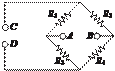### Create an Account

Home / Questions / In the bridge circuit in Figure P266 if nodes or terminals C and D are shorted and V ...

# In the bridge circuit in Figure P266 if nodes or terminals C and D are shorted and V S R1 R3 R6 R7 R8 R1 22 kQ R2 18 kQ R3 47 kQ

In the bridge circuit in Figure P2.66, if nodes (or terminals) and are shorted and

 V

 -

S     +       R1

R3              R6

RR8

R1 = 2.2 kQ          R2 = 18 kQ

R3 = 4.7 kQ          R4 = 3.3 kQ

determine the equivalent resistance between the nodes or terminals and B.

Figure P2.63

2.64      In the circuit of Figure P2.64, find the equivalent resistance looking in at terminals and if terminals and are open and again if terminals and are shorted together. Also, find the equivalent resistance looking in at terminals and if terminals and are open and if terminals and are shorted together.Figure P2.66

Jun 19 2020 View more View LessSubscribe To Get Solution